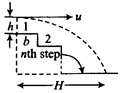Projection Under uniform Acceleration
Question

# A ball rolls off the top of a staircase with a horizontal velocity u ms-1. If the steps are h metre high and b metre wide, the ball will hit the edge of the nth step, if

Moderate
Solution

## If the ball hits the nth step, then horizontal distance traversed = nh.  Here, velocity along horizontal direction $=\mathrm{u}·\mathrm{n}=\mathrm{ut}$ Initial velocity along vertical direction is 0.Get Instant Solutions